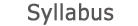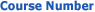0509-1647 Calculus 2b for Mechanical Engineering2019 - 2020View groups
Course description

Background: differential and integral calculus 1b, linear algebra.

1. Limit and continuity of functions of two variables, iterated limits, partial derivatives, the chain rule, changing the order of differentiation, implicit functions and their derivatives. Taylor formula, extremum, Lagrange multiplier method.
2. Double and triple integrals, variables changing in double and triple integrals, Jacobian. Polar, cylindrical, and spherical coordinates. Application in calculation of lengths, areas, volumes, moments and centers of mass.
3. Line integrals of the first and second kinds. Green's theorem. Surface integrals of the first and second kinds.
4. Theory of vector fields, operators: gradient, divergence and rotor. Theorems of Gauss and Stokes.

accessibility declaration